Math resources Geometry

3D shapes

Cylinder

# Cylinder

Here you will learn about cylinders, including how to identify a cylinder based on its attributes and how to identify a cylinder within a composite shape.

Students will first learn about cylinders as part of geometry in 1 st grade.

In middle school and high school, students will expand their knowledge of cylinders when they learn to find the volume of a cylinder as well as the surface area of a cylinder, along with other three dimensional shapes.

## What is a cylinder?

A cylinder is a three dimensional shape, or 3D shape. It has two ends that are both circles of the same size. It has one curved side that goes all the way around.

Properties of a cylinder

A cylinder, like all 3D shapes, can be identified by its properties – the number of faces, edges, and vertices.

Face: a flat surface

A cylinder has two faces. They are the circular ends of the cylinder, just like the tops and bottoms of cans or cups. It also has one curved surface, which can also be considered a face. Note: this is also the base of a cylinder.

Vertices: the corners or points where edges meet

A cylinder doesn’t have any vertices since it has no corners or points.

Edge: the line where two faces of a shape meet

A cylinder has two edges. They are the lines where the curved part of the cylinder meets the circular ends.

Real-life examples of a cylinder

Note: The following content will not apply until middle school.

## Volume of a cylinder

The volume of a cylinder is the amount of space inside a cylinder. The volume of a cylinder is measured in cubic units. You will need to know the height of the cylinder and the radius of the base to find the volume.

The formula for the volume of a cylinder is:

\text{Volume}= \pi r^2 h

Note that r = radius of a cylinder and h = height of a cylinder.

For example, find the volume of the cylinder.

\begin{aligned} \text{Volume}&= \pi r^2 h\\\\ &=\pi \times 4^2 \times 10\\\\ &=160\pi\\\\ &=502.7 \ cm^3 \ \text{(to 1 dp)} \end{aligned}

Step by step guide: Volume of a cylinder

## Surface area of a cylinder

The surface area of a cylinder is the area which covers the outer surface of a cylinder. The surface area of a cylinder is made up of three parts: a curved surface area and two circular bases. The surface area of a cylinder is measured in square units.

The formula for calculating the curved surface area of a cylinder is:

\text{Curved surface area}=2\pi rh

The formula for calculating the area of a circle is:

\text{Area of a circle}=\pi r^2

For the total surface area, we can add the curved surface area to the total area of the bases.

\text{Total surface area}=2\pi rh+2\pi r^2

For example, find the surface area of the cylinder.

\text{Curved surface area}=2\pi rh=2\times \pi \times 4 \times 10=80\pi

\text{Area of a circle}=\pi r^2=\pi \times 4^2=16\pi

\begin{aligned} \text{Total surface area}&=80\pi +2\times 16\pi \\\\ &=80\pi+32\pi\\\\ &=112\pi\\\\ &=315.9 \ cm^2 \ \text{(to 1 dp)} \end{aligned}

Step by step guide: Surface area of a cylinder

### What is cylinder?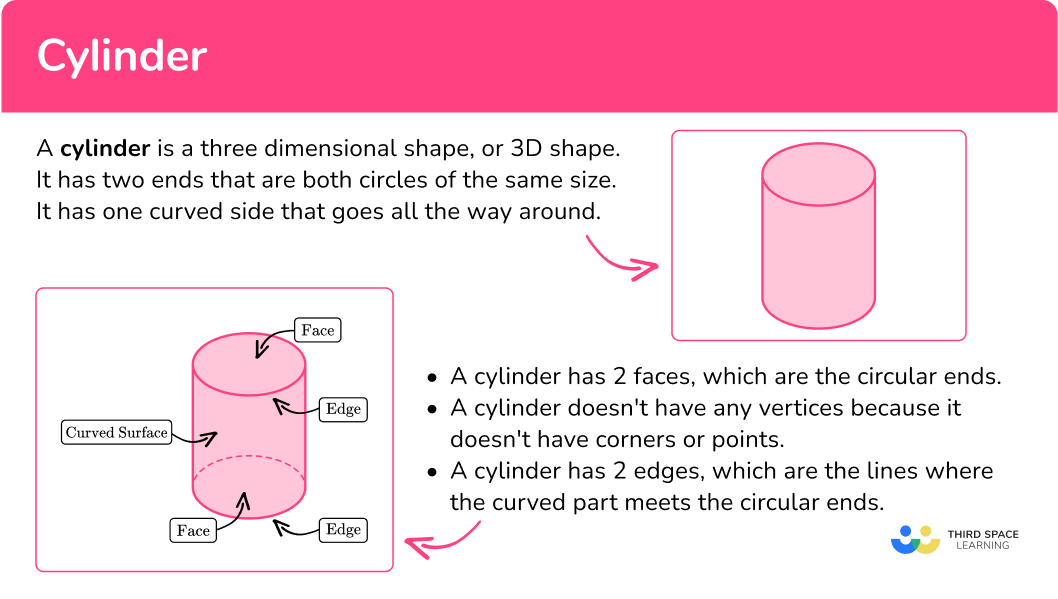## Common Core State Standards

How does this relate to 1 st grade math?

• Grade 1 – Geometry (1.G.2)
Compose two-dimensional shapes (rectangles, squares, trapezoids, triangles, half-circles, and quarter-circles) or three-dimensional shapes (cubes, right rectangular prisms, right circular cones, and right circular cylinders) to create a composite shape, and compose new shapes from the composite shape.

## How to identify a cylinder

In order to determine if a shape is a cylinder:

1. Look at the shape and examine its properties.
2. Determine if it has the properties of a cylinder.

3aIf it does, name the shape as a cylinder.

3bIf it does not, describe the difference in properties.

## Cylinder examples

### Example 1: identify a cylinder

Is this shape a cylinder?

1. Look at the shape and examine its properties.

The shape has 1 circular face, 1 vertex, 0 edges, and a curved surface.

2Determine if it has the properties of a cylinder.

A cylinder has 2 circular faces, no vertices, 2 edges, and a curved surface. It has some properties of a cylinder, but not all of them.

3If it does not, describe the difference in properties.

This shape is not a cylinder because it only has 1 face instead of 2 . It also has 0 edges instead of 2 and it has a vertex, while a cylinder has none.

### Example 2: identify a cylinder

Is this shape a cylinder?

The shape has 2 circular faces, no vertices, 2 edges, and a curved surface.

A cylinder has 2 circular faces, no vertices, 2 edges, and a curved surface. It has all of the properties of a cylinder.

This shape is a cylinder.

### Example 3: identify a cylinder

Is this object a cylinder?

The shape has 2 circular faces, no vertices, 2 edges, and a curved surface.

A cylinder has 2 circular faces, no vertices, 2 edges, and a curved surface. It has all of the properties of a cylinder.

This object is a cylinder.

## How to identify a cylinder in a composite shape

In order to identify cylinders within composite shapes:

1. Look at all the shapes within the composite shape and examine their properties.
2. Determine if any of the shapes have the properties of a cylinder. If they do, identify them.

### Example 4: identify cylinders within composite shapes

There is one cylinder in this composite shape. What color is it?

There are three shapes within the composite shape. The red shape is rectangular and has 6 faces, 12 edges, and 8 vertices.

The yellow shape has 2 circular faces, no vertices, 2 edges, and a curved surface. The green shape has 1 circular face, 1 vertex, 0 edges, and a curved surface.

The yellow shape in the middle has the same properties of a cylinder.

The cylinder is yellow.

### Example 5: identify cylinders within composite shapes

How many cylinders are in this composite shape?

There are 5 shapes in this composite shape.

• Blue and green shapes: These shapes are rectangular; they each have 6 faces, 12 edges, and 8 vertices.

• Orange and red shapes: These shapes each have 2 circular faces, no vertices, 2 edges, and a curved surface.

• Purple shape: The purple shape has 1 circular face, 1 vertex, 0 edges, and a curved surface.

The orange shape and the red shape have the properties of a cylinder.

There are 2 cylinders in this composite shape.

### Example 6: identify cylinders within composite shapes

How many cylinders are in this composite shape?

There are 8 shapes in this composite shape:

• Blue, purple, red shapes: These shapes are rectangular; they each have 6 faces, 12 edges, and 8 vertices.

• Light blue shapes: These shapes each have 2 circular faces, no vertices, 2 edges, and a curved surface.

• Yellow shape: The yellow shape has 1 circular face, 1 vertex, 0 edges, and a curved surface.

The yellow shapes have the properties of a cylinder.

There are 2 cylinders in this composite shape.

## How to find the volume of a cylinder

In order to find the volume of a cylinder:

1. Write down the formula.
2. Substitute the given values.
3. Work out the calculation.
4. Write the final answer, including units.

### Example 7: find the volume of a cylinder

Find the volume of the cylinder with radius 3.8 \, cm and perpendicular height 6.1 cm.

\text { Volume } =\pi r^{2} h

\begin{aligned}\text { Volume } &=\pi r^{2} h \\\\ &=\pi \times 3.8^{2} \times 6.1\end{aligned}

\begin{aligned}&=\pi \times 3.8^{2} \times 6.1 \\\\ &=276.724 \ldots\end{aligned}

=276.7 \mathrm{~cm}^{3} \; (\text {to } 1 . d . p)

## How to find the surface area of a cylinder

In order to find the surface area of a cylinder:

1. Find the area of each face.
3. Include units.

### Example 8: find the surface area of a cylinder

Find the total surface area of the cylinder with radius 7.8 \, cm and perpendicular height 6.5 \, cm. Give your answer to the nearest tenth.

\begin{aligned} \text{Curved surface area}&=2\pi rh\\\\ &=2 \times \pi \times 7.8\times 6.5\\\\ &=318.5574… \ \end{aligned}

\begin{aligned}\text{Area of circle }&=\pi r^2\\\\ &=\pi \times 7.8^2\\\\ &=191.1344…\end{aligned}

Total surface area: 318.5574+191.1344+191.1344=700.8262

Total surface area = 700.8 {~cm^2}

### Teaching tips for cylinder

• Begin by showing real-life examples of cylinders to the students, such as a soda can, a toilet paper roll, or a flashlight. Discuss their shape and point out the circular ends and curved surfaces.

• Provide students with different objects that are cylinders, such as empty paper towel rolls or plastic cups, and let them explore and manipulate these objects. Encourage them to touch and feel the circular ends and the curved surface.

• Utilize visual aids, such as pictures, diagrams, or posters, to visually represent cylinders and their properties. Display images of different cylinders and ask students to identify the circular ends and curved surface.

### Easy mistakes to make

• Confusing cylinders with other shapes
Students may mistakenly identify other shapes, such as cones or prisms, as cylinders. Encourage them to focus on the specific properties of a cylinder, including the circular ends and the curved surface.

• Not recognizing the curved surface
Students might overlook the curved surface of a cylinder and only focus on the circular ends. Emphasize that a cylinder has a smooth, rounded surface that connects the two ends.

• Miscounting the faces
Some students might count only one face on a cylinder instead of recognizing that there are two circular faces. Reinforce the idea that each circular end is a separate face.

• Forgetting the edges
Students may forget to count the edges of a cylinder. Remind them that a cylinder has two edges—the top edge where the curved surface meets the top circle, and the bottom edge where the curved surface meets the bottom circle.

• Thinking that a hollow cylinder is not a cylinder or that it has no volume
A hollow cylinder, such as a toilet paper roll, is still a cylinder even though it is empty and the bases of the cylinder are not seen. Middle school students may think a cylinder such as an empty pringles can has no volume since there is nothing inside. This is not true and both of these examples are still cylinders.

### Practice cylinder questions

1. Which shape is a cylinder?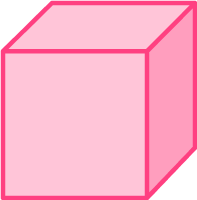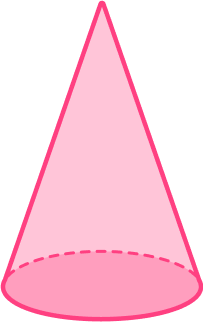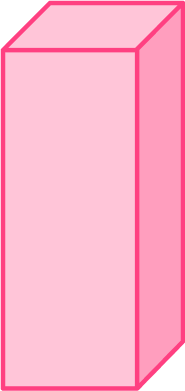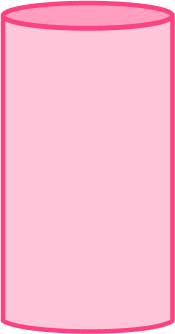A cylinder has 2 circular faces, no vertices, 2 edges, and a curved surface.

The last shape has all of these properties and the others do not.

2. Which shape is NOT a cylinder?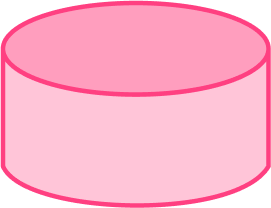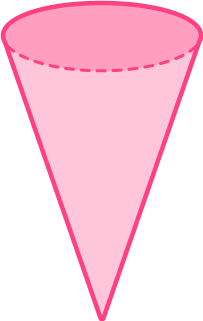A cylinder has 2 circular faces, no vertices, 2 edges, and a curved surface.

All shapes except the second shape, which is a cone, have these properties.

3. How many cylinders are in the composite shape?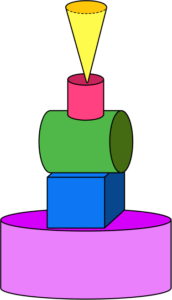3214A cylinder has 2 circular faces, no vertices, 2 edges, and a curved surface.

The 2 nd shape from the top (pink), 3 rd from the top (green), and the bottom shape (purple) have all of these properties.

4. What color is the cylinder in the composite shape?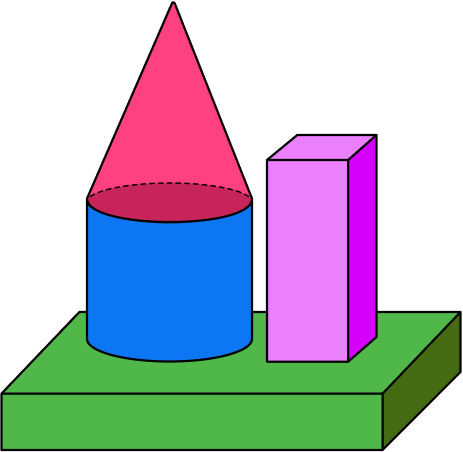redbluepurplegreenA cylinder has 2 circular faces, no vertices, 2 edges, and a curved surface.

The blue shape has all of these properties.

### Middle school practice cylinder questions

5. Find the volume of a cylinder of radius 2.9 \, cm and perpendicular height 5.7 \, cm. Round your answer to the nearest whole number.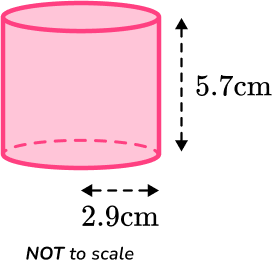150 {~cm^3}151 {~cm^3}296 {~cm^3}297 {~cm^3}You are finding the volume of a cylinder, so you substitute the values of r and h into the formula.

\begin{aligned}V&=\pi r^2 h\\\\ V&=\pi \times 2.9^2 \times 5.7\\\\ V&=150.598…\\\\ V&=151 \, cm^3 \ \text{(to the nearest whole number)}\\\end{aligned}

6. Find the curved surface area of a cylinder.

The radius of the cylinder is 9.5 \, cm and the perpendicular height of the cylinder is 7.2 \, cm. Round your answer to the nearest whole number.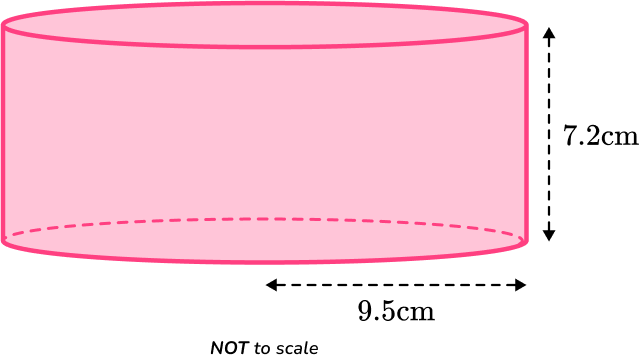430 {~cm^2}428 {~cm^2}429 {~cm^2}427 {~cm^2}You are finding the curved surface area of a cylinder, so you substitute the values of r and h into the formula.

\begin{aligned}\text{Curved surface area}&=2\pi rh\\\\ &=2 \times \pi \times 9.5\times7.2\\\\ &=429.769…\\\\ &=430 \, cm^2 \ \text{(to 3 sf)}\\\end{aligned}

## Cylinder FAQs

What is a cylinder?

A cylinder is a three dimensional shape, or 3D geometric shape. It is a solid figure that has two ends that are both circles of the same size. It has one curved side that goes all the way around.

What are the properties of a cylinder?

A cylinder has 2 circular faces (which are the bases of the cylinder), a curved surface area, 2 edges, and zero vertices.

What is the surface area of a cylinder?

The surface area of a cylinder is the total area of all its surfaces.

How do you find the total surface area of a cylinder?

To find the total surface area of the cylinder, you need to add the areas of the two circular bases and the area of the curved surface:

\begin{aligned}\text{Total Surface Area }&=2 \times (\text{Area of Base})+(\text{Area of Curved Surface})\\\\ & =2 \times \pi r^2+2 \pi r h \\\\ & =2 \pi r(r+h)\end{aligned}

Are there different types of cylinders?

Yes, there are different types of cylinders, including a right cylinder, oblique cylinder, elliptic cylinder and right circular hollow cylinder.

What is the axis of a cylinder?

The axis of the cylinder is an imaginary straight line that passes through the center of the cylinder and is parallel to the circular bases. It is the line segment around which the cylinder can be rotated to create a symmetrical shape.

## Still stuck?

At Third Space Learning, we specialize in helping teachers and school leaders to provide personalized math support for more of their students through high-quality, online one-on-one math tutoring delivered by subject experts.

Each week, our tutors support thousands of students who are at risk of not meeting their grade-level expectations, and help accelerate their progress and boost their confidence.